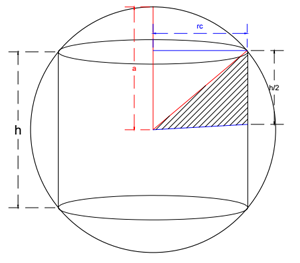### Math Notes

Subjects

#### Differential Calculus Solutions

##### Topics || Problems

A cylinder is inscribed in a given sphere of radius a. Find the dimension of the cylinder if its lateral surface area is maximum.

Solution:The lateral surface area of the cylinder is $$A=2\pi r_c h$$:

Since the radius of the sphere is a constant, "$$a$$", rewrite the lateral surface area of the cylinder as a function of the its height or its radius with the radius of the sphere ($$a$$).

$$(\frac{h}{2})^2 +(r_c)^2=a^2$$

$$r_c = \frac{1}{2} \sqrt{4a^2-h^2}$$

$$A=2\pi r_c h$$

$$A=2\pi (\frac{1}{2} \sqrt{4a^2-h^2}) h$$

$$A=\pi (\sqrt{4a^2-h^2}) \sqrt{h^2}$$

$$A=\pi \sqrt{4h^2a^2-h^4}$$: The lateral surface area of the cylinder as a function of $$h$$ and $$a$$

Maximize the lateral surface area $$A$$.

$$\frac{dA}{dh}=\pi \frac{1}{2}\frac{8ha^2-4h^3}{\sqrt{4h^2a^2-h^4}}$$

$$0=\pi \frac{1}{2}\frac{8ha^2-4h^3}{\sqrt{4h^2a^2-h^4}}$$

$$0=8ha^2-4h^3$$

$$h^2=2a^2$$

$$h=a\sqrt{2}$$

$$r_c=a\frac{\sqrt{2}}{2}$$

Thus the dimension of the cylinder (at maximum lateral surface area) inside a sphere of radius $$a$$ is:

$$h=a\sqrt{2}$$

$$r_c=a\frac{\sqrt{2}}{2}$$